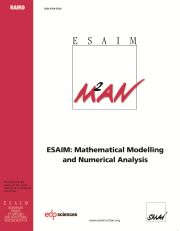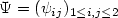Home
Hostname: page-component-684899dbb8-662rr Total loading time: 0.196 Render date: 2022-05-20T11:49:06.578Z Has data issue: true Feature Flags: { "shouldUseShareProductTool": true, "shouldUseHypothesis": true, "isUnsiloEnabled": true, "useRatesEcommerce": false, "useNewApi": true }ESAIM: Mathematical Modelling and Numerical Analysis

# Isoparametric mixed finite element approximation of eigenvalues and eigenvectors of 4th order eigenvalue problems with variable coefficients

Published online by Cambridge University Press:  15 April 2002

## Abstract

Estimates for the combined effect of boundary approximation and numerical integration on the approximation of (simple) eigenvalues and eigenvectors of 4th order eigenvalue problems with variable/constant coefficients in convex domains with curved boundary by an isoparametric mixed finite element method, which, in the particular case of bending problems of aniso-/ortho-/isotropic plates with variable/constant thickness, gives a simultaneous approximation to bending moment tensor field $\Psi= (\psi_{ij})_{1 \le i,j \le 2}$and displacement field `u', have been developed.

Type
Research Article
Information

## Access options

Get access to the full version of this content by using one of the access options below. (Log in options will check for institutional or personal access. Content may require purchase if you do not have access.)

## References

I. Babuska and J.E. Osborn, Eigenvalue Problems, in Handbook of Numerical Analysis. Vol. II, P.G. Ciarlet and J.-L. Lions, Eds., in Finite Element Methods (Part 1), North-Holland Publishing Company, Amsterdam, New York, Oxford (1991) 641-787.
Balasundaram, S. and Bhattacharyya, P.K., On existence of solution of the Dirichlet problem of fourth-order partial differential equations with variable coefficients. Quart. Appl. Math. 39 (1983) 311-317. CrossRef
Balasundaram, S. and Bhattacharyya, P.K., A mixed finite element method for fourth-order elliptic equations with variable coefficients. Comput. Math. Appl. 10 (1984) 245-256. CrossRef
Banerjee, U., A note on the effect of numerical quadrature in finite element eigenvalue approximation. Numer. Math. 61 (1992) 145-152. CrossRef
Banerjee, U. and Osborn, J.E., Estimates of the effect of numerical integration in finite element eigenvalue approximation. Numer. Math. 56 (1990) 735-762. CrossRef
M. Bernadou, Méthodes numériques pour les problèmes elliptiques, in Méthodes de Mathématiques Appliquées, Vol. 1, Chap. XI, C.E.A., France (1981).
P.K. Bhattacharyya and N. Nataraj, Isoparametric Mixed Finite Element Approximation of Eigenvalues and Eigenvectors of 4th-Order Eigenvalue Problems with Variable Coefficients. Research Report No. R 98021, Laboratoire d' Analyse Numérique, Université de Paris VI (1998).
Bhattacharyya, P.K. and Nataraj, N., Combined Effect, On of Boundary Approximation and Numerical Integration on Mixed Finite Element Solution of 4th order Elliptic Problems with Variable Coefficients. ESAIM: M2AN 133 (1999) 807-836. CrossRef
P.K. Bhattacharyya and N. Nataraj, Error Estimates for Isoparametric Mixed Finite Element Solution of 4th-Order Elliptic Problems with Variable Coefficients. In preparation.
Brezzi, F., On the existence, uniqueness and approximation of saddle-point problems arising from Lagrangian multipliers. RAIRO Anal. Numér. 8 (1974) 129-151.
F. Brezzi and M. Fortin, Mixed and Hybrid Finite Element Methods. Springer-Verlag, Berlin, Heidelberg, New York (1991).
F. Brezzi and P.A. Raviart, Mixed finite element methods for 4th-order elliptic equations. Topics Numer. Anal. III , J. Miller, Ed., Academic Press, New York (1978) 33-56.
Canuto, C., Eigenvalue approximation by mixed methods. RAIRO Anal. Numér. 12 (1978) 27-50. CrossRef
F. Chatelin, Spectral Approximation of Linear Operators. Academic Press, New York (1983).
P.G. Ciarlet, The Finite Element Method for Elliptic Problems. North-Holland Publishing Company, Amsterdam, New York, Oxford (1978).
P.G. Ciarlet and P.A. Raviart, The combined effect of curved boundaries and numerical integration in isoparametric finite element methods, in Math. Found. Finite El. Method Appl. Part. Differ. Equations, A.K. Aziz and I. Babuska, Eds., Sympos. Univ. Maryland, Baltimore (1972) 409-474.
M. Dauge, Elliptic Boundary Value Problems on Corner Domains. Springer-Verlag, Berlin, Heidelberg, New York (1988).
N.J. DeCapua and B.C. Sun, Transverse vibration of a class of orthotropic plates. J. Appl. Mech. (1972) 613-615.
Gopalsamy, S. and Bhattacharyya, P.K., On existence and uniqueness of solution of boundary value problems of fourth-order elliptic partial differential equations with variable coefficients. J. Math. Anal. Appl. 136 (1988) 589-608.
P. Grisvard, Elliptic Problems in Nonsmooth Domains. Pitman, Boston (1985).
Ishihara, K., A mixed finite element method for the biharmonic eigenvalue problem of plate bending. Publ. Res. Inst. Math. Sci. Kyoto Univ. 14 (1978) 399-414. CrossRef
Ishihara, K., The buckling of plates by the mixed finite element method. Mem. Numer. Math. 5 (1978) 73-82.
Kondratev, V.A., Boundary value problems for elliptic equations in domains with conical or angular points. Trudy Moskov. Mat. Obshch. 16 (1967) 209-292.
Lebaud, M.P., Error estimate in an isoparametric finite element Eigenvalue Problem. Math. Comp. 63 (1994) 19-40. CrossRef
A.W. Leissa, Vibration of Plates. NASA SP-160 (1969).
S.G. Leknitskii, Anisotropic Plates. Gordon and Breach Science Publishers, New York (1968).
J.-L. Lions, Problèmes aux limites dans les équations aux dérivées partielles. Presses Univ. Montréal, Montreal (1965).
Mercier, B., Osborn, J., Rappaz, J. and Raviart, P.A., Eigenvalue approximation by mixed and hybrid methods. Math. Comp. 36 (1981) 427-453. CrossRef
Miyoshi, T., A finite element method for the solution of fourth-order partial differential equations. Kumamoto J. Sci. (Math.) 9 (1973) 87-116.
N. Nataraj, On Mixed Finite Element Analysis Of Fourth Order Elliptic Source/Eigenvalue Problems in Convex Domains. Ph.D. Thesis, Dept. of Mathematics, Indian Institute of Technology, Delhi (1998).
L.A. Oganesian and L.A. Rukhovec, Variational difference methods for the solution of elliptic problems. Izv. Akad. Nauk Armyan. SSR, Yerevan (1979). In Russian.
A.B. Ouaritini, Méthodes d'éléments finis mixtes pour des problèmes de coques minces. Thèse de Docteur de 3 e cycle, Université de Pau et des Pays de L'Adour, France (1984).
O. Pironneau, Méthodes des éléments finis pour les fluides. Masson, Paris (1988).
P.A. Raviart and J.M. Thomas, Introduction à l'analyse numérique des équations aux dérivées partielles. Masson, Paris (1983).
J.E. Roberts and J.M. Thomas, Mixed and Hybrid Methods, in Handbook of Numerical Analysis. Vol. II, in Finite Element Methods (Part 1), P.G. Ciarlet and J.-L. Lions, Eds., North-Holland Publishing Company, Amsterdam, New York, Oxford, (1991) 523-633.
G. Strang and G.J. Fix, An Analysis of the Finite Element Method. Prentice-Hall, New York (1973).
S. Timoshenko and S. Woinowsky-Kreiger, Theory of Plates and Shells. McGraw-Hill Book Company, New York (1959).
Vanmaele, M. and Zenísek, A., External finite-element approximations of eigenvalue problems. RAIRO Modél. Math. Anal. Numér. 27 (1993) 565-589. CrossRef
M. Vanmaele and A. Zenísek, The combined effect of numerical integration and approximation of boundary in the finite element method for eigenvalue problems. Numer. Math. (1995).
A. Zenísek, Nonlinear Elliptic and Evolution Problems and their Finite Element Approximations. Academic Press, New York (1990).

# Save article to Kindle

Note you can select to save to either the @free.kindle.com or @kindle.com variations. ‘@free.kindle.com’ emails are free but can only be saved to your device when it is connected to wi-fi. ‘@kindle.com’ emails can be delivered even when you are not connected to wi-fi, but note that service fees apply.

Find out more about the Kindle Personal Document Service.

Isoparametric mixed finite element approximation of eigenvalues and eigenvectors of 4th order eigenvalue problems with variable coefficients
Available formats
×

# Save article to Dropbox

To save this article to your Dropbox account, please select one or more formats and confirm that you agree to abide by our usage policies. If this is the first time you used this feature, you will be asked to authorise Cambridge Core to connect with your Dropbox account. Find out more about saving content to Dropbox.

Isoparametric mixed finite element approximation of eigenvalues and eigenvectors of 4th order eigenvalue problems with variable coefficients
Available formats
×

# Save article to Google Drive

To save this article to your Google Drive account, please select one or more formats and confirm that you agree to abide by our usage policies. If this is the first time you used this feature, you will be asked to authorise Cambridge Core to connect with your Google Drive account. Find out more about saving content to Google Drive.

Isoparametric mixed finite element approximation of eigenvalues and eigenvectors of 4th order eigenvalue problems with variable coefficients
Available formats
×
×